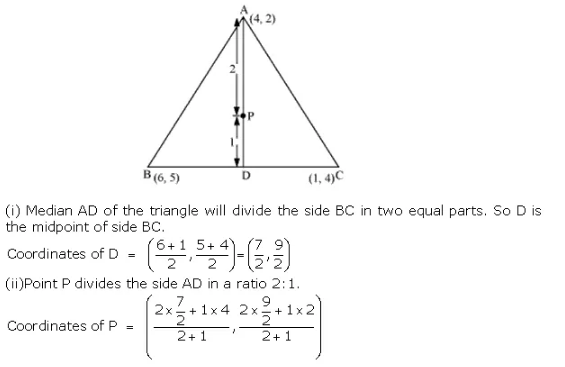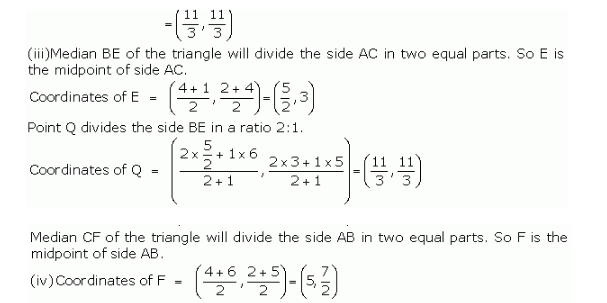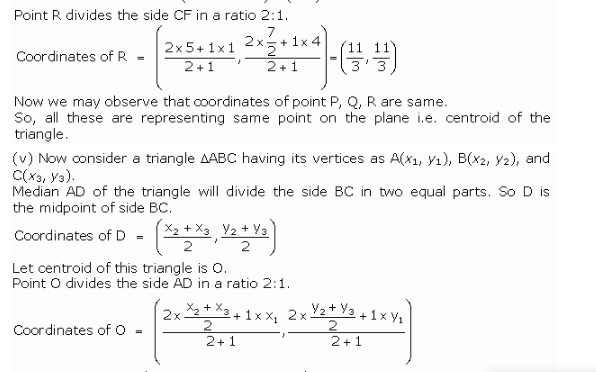• +91 9971497814
• info@interviewmaterial.com

# Coordinate Geometry Ex-7.4 Interview Questions Answers

### Related Subjects

Question 1 :

Determine the ratioin which the line 2x + y – 4 = 0 divides the line segment joining the pointsA(2, –2) and B(3, 7).

Consider line 2x + y – 4 = 0 divides line ABjoined by the two points A(2, -2) and B(3, 7) in k : 1 ratio.

Coordinates of point of division can be givenas follows:

x = and y =

Substituting the values of x and y givenequation, i.e. 2x + y – 4 = 0, we have

2( + (– 4 = 0

+ ( = 4

4 + 6k – 2 + 7k = 4(k+1)

-2 + 9k = 0

Or k = 2/9

Hence, the ratio is 2:9.

Question 2 : Find the relation between x and y if thepoints (x, y), (1, 2) and (7, 0) are collinear.

If given points are collinear then area oftriangle formed by them must be zero.

Let (x, y), (1, 2) and (7, 0) are vertices ofa triangle,

Area of a triangle = = 0

[x(2 – 0) + 1 (0 – y) + 7( y– 2)] = 0

• 2x – y + 7y – 14 = 0
• 2x + 6y – 14 = 0
• x + 3y – 7 = 0. Which is required result.

Question 3 :

Find the centre of acircle passing through points (6, -6), (3, -7) and (3, 3).

Let A = (6, -6), B = (3, -7), C = (3, 3) arethe points on a circle.

If O is the centre, then OA = OB = OC (radiiare equal)

If O = (x, y) then

OA =

OB =

OC =

Choose: OA = OB, we have

After simplifying above, we get -6x = 2y – 14….(1)

Similarly: OB = OC

(x – 3)+ (y + 7)2 =(x – 3)2 + (y – 3)2

(y + 7)2 = (y – 3)2

y+ 14y + 49 = y–6y + 9

20y =-40

or y = -2

Substituting the value of y in equation (1),we get;

-6x = 2y – 14

-6x = -4 – 14 = -18

x = 3

Hence, centre of the circle located at point(3,-2).

Question 4 :

The two oppositevertices of a square are (-1, 2) and (3, 2). Find the coordinates of the othertwo vertices.

Answer 4 :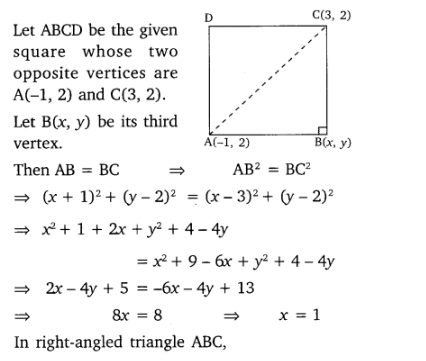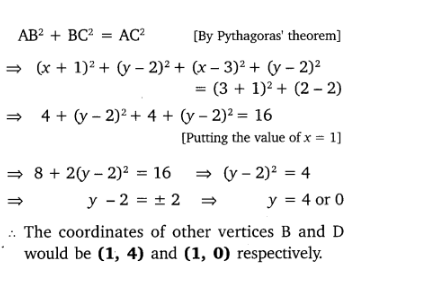Question 5 :

The class X studentsof a secondary school in Krishinagar have been allotted a rectangular plot ofland for their gardening activity.

Saplings of Gulmohar are planted on the boundary at a distance of 1 m from each other. There is a triangular lawn in the plot as shown in the fig. 7.14. The students are to sow the seeds of flowering plants on the remaining area of the plot.

(i) Taking A as origin, find the coordinates of the vertices of the triangle.

(ii) What will be the coordinates of the vertices of triangle PQR if C is the origin?

Also calculate the areas of the triangles in these cases. What do you observe?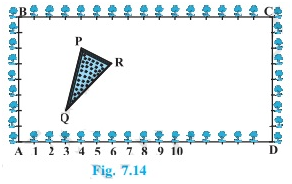Solution:

(i) Taking A as origin, coordinates of thevertices P, Q and R are,

From figure: P = (4, 6), Q = (3, 2), R (6, 5)

Here AD is the x-axis and AB is the y-axis.

(ii) Taking C as origin,

Coordinates of vertices P, Q and R are ( 12,2) , (13, 6) and (10, 3) respectively.

Here CB is the x-axis and CD is the y-axis.

Find the area of triangles:

Area of triangle PQR in case of origin A:

Using formula: Area of a triangle =

= ½ [4(2 – 5) + 3 (5 – 6) + 6 (6 – 2)]

= ½ ( – 12 – 3 + 24 )

= 9/2 sq unit

(ii) Area of triangle PQR in case of origin C:

Area of a triangle =

= ½ [ 12(6 – 3) + 13 ( 3 – 2) + 10( 2 – 6)]

= ½ ( 36 + 13 – 40)

= 9/2 sq unit

This implies, Area of triangle PQR at origin A= Area of triangle PQR at origin C

Area is same in both case because triangleremains the same no matter which point is considered as origin.

Question 6 :

The vertices of a ∆ABC are A (4, 6), B (1, 5) and C (7, 2). A line is drawn to intersect sides ABand AC at D and E respectively, such that . Calculate the area of the ∆ ADE andcompare it with area of ∆ ABC. (Recall Theorem 6.2 and Theorem 6.6)

Answer 6 :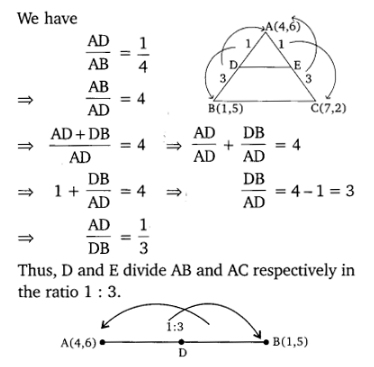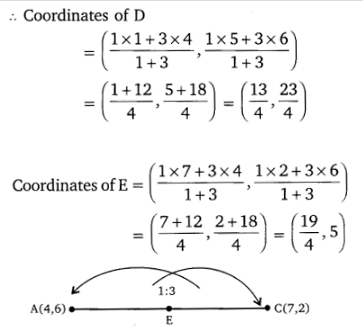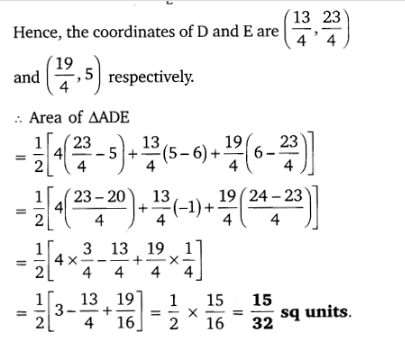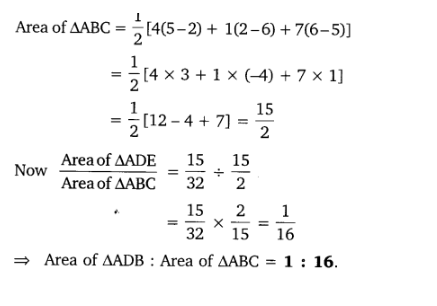Question 7 : Let A (4, 2), B (6, 5) and C (1, 4) be the vertices of ∆ ABC.

(i) The median fromA meets BC at D. Find the coordinates of point D.

(ii) Find thecoordinates of the point P on AD such that AP : PD = 2 : 1.

(iii) Find thecoordinates of point Q and R on medians BE and CF respectively such that BQ :QE = 2:1 and CR : RF = 2 : 1.

(iv) What do youobserve?

[Note : The pointwhich is common to all the three medians is called the centroid

and this pointdivides each median in the ratio 2 : 1.]

(v) If A (x1,y1), B (x2, y2) and C (x3, y3)are the vertices of triangle ABC, find the coordinates of the centroid of thetriangle.

Solution